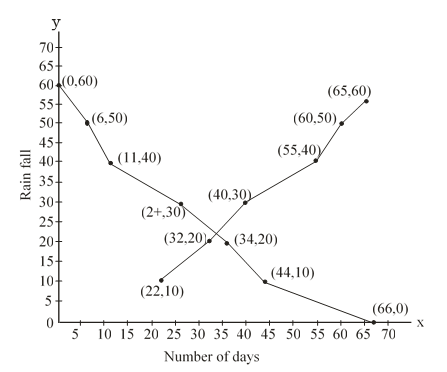#### The annual rainfall record of a city for 66 days is given in the following table. Rainfall (in cm) 0-10 10-20 20-30 30-40 40-50 50-60 Number of days 22 10 8 15 5 6 Calculate the median rainfall using ogives (of more than type and of less than type)Solution.

 (i) less than type (ii) more than type Rain fall No.of days Rain fall Number of days less than 0 0 more than or equal to 0 66 less than 10 0+22 = 22 more than or equal to 10 66-22 = 44 less than 20 22+10 = 32 more than or equal to 20 44-10 = 34 less than 30 32+8 = 40 more than or equal to 30 34-8 = 26 less than 40 40+15 = 55 more than or equal to 40 26-15 = 11 less than 50 55+5 =60 more than or equal to 50 11 - 5 =6 less than 60 60+6 =66 more than or equal to 60 6-6 =0

Now let us draw ogives of more than type and of less than type then find the medianHere median is 20